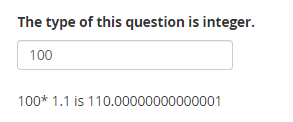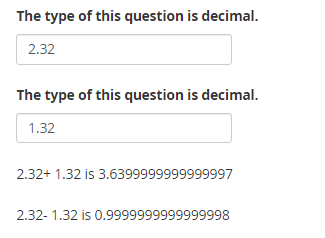## Description

Calculate type question behaves differently on certain numbers and creates unnecessary decimal digits.

## Steps to Reproduce

A)

1. Create an `integer` type question (Q1)
2. Create a `calculate` question (C1) with a simple `\${Q1} * 1.1` formula.
3. Create a `note` to display `\${C1}`
4. Write 100 to Q1.
5. The `note` displays 110.00000000000001 instead of 110

B)

1. Create two `decimal` questions `Q2` and `Q3`
2. Create a `calculate` question (C4) with a simple `\${Q2} + \${Q3}` formula.
3. Create a note to display `\${C4}`.
4. Write 2.32 and 1.32 to `Q2` and `Q3`.
5. The note displays 3.6399999999999997 instead of 3.64

## Expected behavior

The expected behavior is to `calculate` works same in every number.

## Actual behavior

The `calculate` works different for some numbers. See screenshots for different behaviors:• So far, I’ve tested and confirmed that these numbers create some sort of problem in the following calculation: `integer * 1.1` : “3, 6, 7, 11, 12, 100, 200, 400, 700, 800”

• There is a form where you can check additional calculations:
Enketo Express for KoboToolbox

• There is an xlsform with additional operators. Feel free to check it yourselves:
Calculate Problems.xlsx (10.1 KB)

2 Likes

we can use the round-off.

1 Like

I have done this, but it mess up other number that doesnot need to be round off tho,

1 Like

I don’t think rounding-off always would solve the issue for everyone.

Hi @hakan_cetinkaya , do you find any solution?

@Kal_Lam would you mind suggesting please?

@Okard, Unfortunately not.